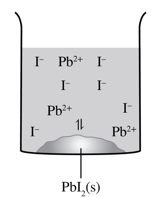# Calculating Solubility and Solubility Product

This topic is part of the HSC Chemistry course under the section Solution Equilibria.

### HSC Chemistry Syllabus

• Derive equilibrium expressions for saturated solutions in terms of Ksp and calculate the solubility of an ionic substance from its Ksp value

• Predict the formation of a precipitate given the standard reference values for Ksp

### How to calculate the solubility product of an equilibrium system?

This video will explore how solubility product is calculated, as well as how the solubility table can be used to predict the formation of a precipitate following an ion-exchange reaction between two solutions.

### Solubility Product Ksp

• Dissolution of ionic compounds forms an equilibrium between dissolved ions and undissolved solids.

• Dissolution of lead (II) fluoride:

$${\rm PbF}_{2(s)}\rightleftharpoons {\rm Pb}_{(aq)}^{2+}+{2F}_{(aq)}^-$$

The expression of equilibrium constant or solubility product for this reaction is:

$$K_{sp}=[{Pb}^{2+}][F^-]^2$$

 The concentration of solids (ionic compounds) is not included in the expression because it stays relatively constant compared to the dissolved ions. The concentrations of dissociated ions are always expressed in mol L-1 (M). Solids and liquids are also heterogenous in that they do not disperse throughout the solution. At equilibrium, the rates of dissolution and re-formation of ionic compound are equal. This is known as the point of saturation as no more ions can dissolve in the solvent. Adding more solutes to a solution at saturation point (equilibrium) will cause precipitation to happen.Figure: Dissociation of solid PbI2 in a beaker.

• Solubility product constant varies between ionic compounds. It indicates how far the dissolution proceeds at equilibrium.
• A large solubility product value indicates high solubility as a relatively large quantity of ions are dissolved at equilibrium.
• Vice versa, a small solubility product value indicates low solubility as relatively small quantity of ions are dissolved at equilibrium.

Table: Solubility product expressions of selected few ionic compounds.

 Ionic compound Dissolution reaction Solubility product Magnesium carbonate $${\rm MgCO}_{3(s)}\rightleftharpoons{\rm Mg}_{(aq)}^{2+}+{\rm CO}_{3(aq)}^{2-}$$ $$K_{sp}=[{\rm Mg}^{2+}][{\rm CO}_3^{2-}]$$ Iron (II) hydroxide $${\rm Fe(OH)}_{2(s)}\rightleftharpoons{\rm Fe}_{(aq)}^{2+}+{\rm 2OH}_{(aq)}^-$$ $$K_{sp}=[{\rm Fe}^{2+}][{\rm OH}^-]^2$$ Calcium phosphate $${{\rm Ca}_3(PO_4)}_{2(s)}\rightleftharpoons{\rm 3Ca}_{(aq)}^{2+}+{\rm 2PO}_{4(aq)}^{3-}$$ $$K_{sp}=[{\rm Ca}^{2+}]^3[{\rm PO}_4^{3-}]^2$$

Example 1

The following table shows the solubility product constants of selected ionic compounds at 25ºC.

 Name, formula Ksp Aluminium hydroxide, Al(OH)3 3 xx 10^(-34) Lead(II) fluoride, PbF2 3.6 xx 10^(-8) Silver sulfide, Ag2S 8.0 xx 10^(-48) Cobalt(II) carbonate, CoCO3 1.0 xx 10^(-10)

Rank the ionic compounds in the table in order of increasing solubility.

Solution: Silver sulfide, aluminium hydroxide, cobalt (II) carbonate, lead(II) fluoride – order of increasing K_{sp}

### Solubility of Ionic Compounds

• The solubility of an ionic compound is usually expressed as the amount of solute dissolved per volume of solvent. This can be either expressed as mass per volume e.g. g/100 mL or as molarity, mol L-1 (M) .
• When solubility of an ionic compound is known, its Ksp can be determined. For example, at 25ºC, the solubility of PbF2 is found to be 0.64 g/L. Calculate the Ksp of PbF2.

 Step 1: Convert solubility into mol L-1 by dividing by the molar mass of the ionic compound. In this case, the molar mass of PbF2 = 245.2 g mol-1 $$n=\frac{0.64\ g}{245.2\ g\ {\rm mol}^{-1}}$$ $$n=0.0026\ mol$$ Therefore, solubility = 0.0026 mol L-1 (M) Step 2: Write an equation representing the dissociation of the ionic compound. $${\rm PbF}_{2(s)}\rightleftharpoons{\rm Pb}_{(aq)}^{2+}+{\rm 2F}_{(aq)}^-$$ Step 3: Write an expression for the solubility product of the ionic compound and find the concentration of each ion. $$K_{sp}=[{\rm Pb}^{2+}][{\rm F}^-]^2$$  $$K_{sp}=[0.0026][2×0.0026]^2$$ $$K_{sp}=7.1\times{10}^{-8}$$

• Using Ksp values from the data sheet, solubility of various ionic compounds can be determined.

 Step 1: Write an equation representing the dissociation of the ionic compound. $${\rm PbI}_{2(s)}\rightleftharpoons{\rm Pb}_{(aq)}^{2+}+{\rm 2I}_{(aq)}^-$$ Step 2: Write an expression for the solubility product of the ionic compound. $$K_{sp}=[{\rm Pb}^{2+}][{\rm I}^-]^2$$ Step 3: Let s be the solubility of the ionic compound. Assign concentration of ions in terms of s. $${\rm PbI}_{2(s)}\rightleftharpoons{\rm Pb}_{(aq)}^{2+}+{\rm 2I}_{(aq)}^-$$             s              s            2s Step 4: Substitute Ksp (from data sheet) and concentrations of ions in terms of s into Ksp expression. $$9.8\times{10}^{-9}=[s][2s]^2$$ Step 5: Solve for solubility (s). $$9.8\times{10}^{-9}=4s^3$$ $$s=1.3\times{10}^{-3} mol L^{-1}$$

## Practice Questions

Determining Ksp from solubility

Question 1

Lead(II) sulfate (PbSO4) is a key component in lead acid car batteries.

(a) Its solubility in water at 25°C is 4.25 xx 10-3 g/100 mL. What is the Ksp of PbSO4?

(b) In terms of their constituent ions, explain why lead(II) fluoride has a much greater solubility than lead(II) sulfate.

Question 2

The solubility of silver chloride in water at 25ºC is 1.34 xx 10-5.What is the Ksp of silver chloride?

Question 3

The solubility of calcium hydroxide in water at 25ºC is 0.074 g/100 mL. What is the Ksp of calcium hydroxide?

Determining solubility from Ksp

Question 1

Ksp of barium sulfate is 11.1 xx 10-10. What is the solubility of barium sulfate in moles per litre? What about in grams per mL?

Question 2

Ksp of iron(III) hydroxide is 14.4 xx 10-38. What is the solubility of iron(III) hydroxide in moles per litre? What about in grams per mL?

Previous section: Dissolution of Ionic Compound

Next section: Calculating Solubility Product Quotient Q